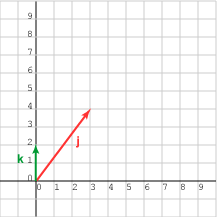Normalize each vector. This does not change the orientations. Now there are two unit vectors and the cosine of the angle between them can be found with the dot product.

# Angle between Non-unit VectorsFind the angle between the two vectors:

j  =  (3, 4)T ,   k  =  (0, 2)T

First, find the length of each vector:

|j|  =  5.0 ,   |k|  =  2.0.

Normalize each vector. Often in this step it is wise not to perform the division:

ju  =  (3, 4)T / 5.0 ,   ku  =  (0, 2)T / 2.0

Compute the dot product:

ju · ku  =  (3, 4)T / 5.0 · (0, 2)T / 2.0  = (1/10)(3, 4)T · (0, 2)T  =  (0.1)(8)  =  0.8.

Finally use the arc cos function of your calculator: arc cos 0.8 =  36.87°

### QUESTION 15:

Calculate the angle between:   a  =  (10, 5)T   and   b  =  (8, 12)T